Cyber Monday Sale: ALL MCAT Products & Admissions Services

MCAT Content / Sound / Production Of Sound

### Production of Sound

Topic: Sound

Sound can be produced in many ways, by many different instruments and devices.

Vibrating Strings
There are many instruments that produce sound based on strings like guitars, cellos, and pianos. These sounds are produced by standing waves in the strings. These waves and their frequencies are constant, and therefore the sound and pitch produced by them is constant. This figure shows a visual of a standing wave in a string: The speed of the wave is proportional to the root of the string tension, and inversely proportional to the root of the string density, shown by the following equation:Pitch, and the way the sound is perceived, depends on the frequency of the sound wave. Using the wave velocity, you can find the frequency using this equation: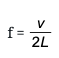where L is the length of the string.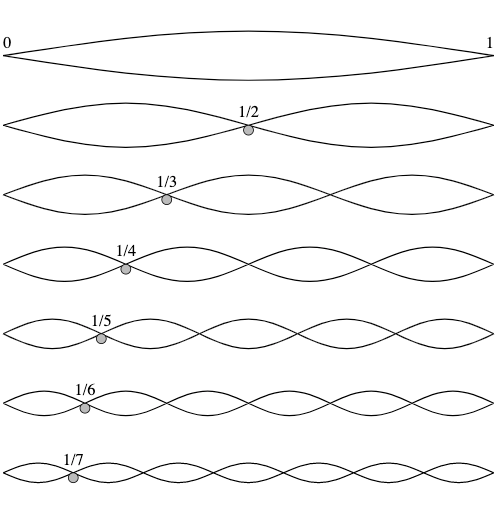Air Columns

Many instruments make music by causing a vibration to a column of air in a resonator. These are usually called wind instruments like flutes, recorders, and saxophones. They are either open at both ends, or closed at one end and open at the other. The pitch of the sound is a function of the frequency. There are many factors that go into finding the frequency. Let’s start with the tubes that are closed at one end and open at the other.

Closed Air Tubes
The maximum displacement of the air occurs at the open end of the tube, and is called the antinode. The air movement is constrained at the closed end, and there is no displacement, and this is called the node. The distance from the node to the antinode is 1/4 the length of one wavelength, and equal to the length of the tube, as shown in this equation:

The frequency is equal to the speed of sound in the air divided by the wavelength, or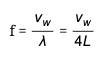where vw is the speed of sound in the air, which we learned how to find in a previous atom.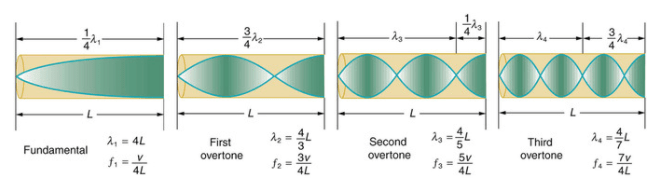Open Air Tubes
Air tubes can also be open at both ends. They are very similar to the ones we talked about above, but there is an antinode at both ends, since they are both open, as shown in this figure: Since there is an antinode at both ends, we can see that the length of a wavelength is found by this equation:

The frequency can be found in the following equation: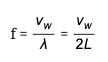Practice Questions

MCAT Official Prep (AAMC)

Official Guide C/P Section Question 28

Official Guide C/P Section Question 29

Key Points

• The frequency, and therefore the pitch of a string instrument depends on the velocity of the sound wave and the length of the string. The frequency is found by: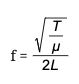Sound in a tube of air that is closed at one end is found by the following equation:• Sound in a tube of air that is open at one end is found by the following equation:Key Terms

node: Point on a wave where there is no displacement.

antinode: A region of maximum amplitude situated between adjacent nodes of a vibrating body, such as a string

Billing Information